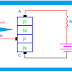## SCR Triggering / SCR Turn On Method

SCR is unidirectional, reverse blocking semiconductor device. Today we are going to learn SCR Triggering Methods or you can say SCR Turn On Methods. Because we already know that SCR cannot conduct current in forward condition until we manually turn it on.

### What is SCR Triggering?

The process of switching the SCR from OFF state to ON state is called SCR Triggering. That means the Turn ON of SCR is also known as SCR Triggering.
In other words, we can say the process of switching the SCR from forward blocking state to forward conduction state is called SCR Triggering.

### Methods of SCR Triggering:

There are many methods of SCR turn on or triggering. The methods of SCR triggering are,

1. Thermal or Temperature Triggering
3. Voltage Triggering or Breakover Voltage Turn On
4. dv/dt triggering
5. Gate Triggering

### What is the Thermal or Temperature Triggering of SCR?

We already know that when the anode of the SCR is positive respect to the cathode the junctions J1 and J2 will be the forward bias and J2 will be the reverse bias. So a small amount of reverse leakage current will flow across the junction J2.

As the leakage current depends on the temperature of the junction, in fact, it is directly proportional to the junction temperature. So when the reverse leakage current flow through the junction the temperature will increase which further increase the flow of current. So when the value of the current increased greater than the Latching Current the SCR will be Turn ON.

### What is the Radiation or Light Triggering of SCR?

If a beam of light is applied to the junction of the SCR it can produce sufficient energy to break the electron-hole pairs in the semiconductor and the SCR will get Turn ON.

So when the intensity of the applied light is increased more than a nominal value the SCR start conducting. It is not an easy process that is why it is used rarely. This triggering mainly used in HVDC Transmission Lines.

### What is the Voltage Triggering or Breakover Voltage Turn On of SCR?

In forward condition the junction J1 and J3 are forward biased and the junction J2 is reverse biased. So if we add an additional voltage greater than the normal rated forward voltage across the SCR the Junction J2 will go to avalanche breakdown.

So the current will start flowing through the junction J2. And also the current will flow through the whole SCR. This additional applied voltage is known as Breakover Voltage. Remember that in this case, the anode current would be limited by only the external load impedance.

### What is the dv/dt triggering of SCR?

We know that in forward condition the junction J1 and J3 are forward biased and the junction J2 is reverse biased. So we can assume that there is a capacitance will produce in the SCR whose junction J2 act as a dielectric medium and the upper portion of the junction J2 is connected to the positive voltage and lower portion to the negative voltage.

In this situation, if we increase the rate of change in forward anode-cathode voltage a transient gate current will produce(even the gate terminal is open) which can turn on the SCR.
As in this method, we create change the voltage respect to the time it is called dv/dt triggering.

### What is the Gate triggering of SCR?

The process of addition minority carriers into the gate region through the gate electrode to switch on the SCR is known as Gate Triggering.
If we apply a positive voltage to the gate with respect to the cathode a current will flow through the gate terminal and if the gate current is large enough the SCR will switch ON.

### Various methods of Gate triggering of SCR:

1. Triggering by a DC Gate Signal
2. Triggering by an AC Gate Signal
3. Triggering by a Pulsed Gate Signal

SCR Triggering / SCR Turn On Methods Full explanation.Reviewed by manoranjan das on 11:27 am Rating: 5